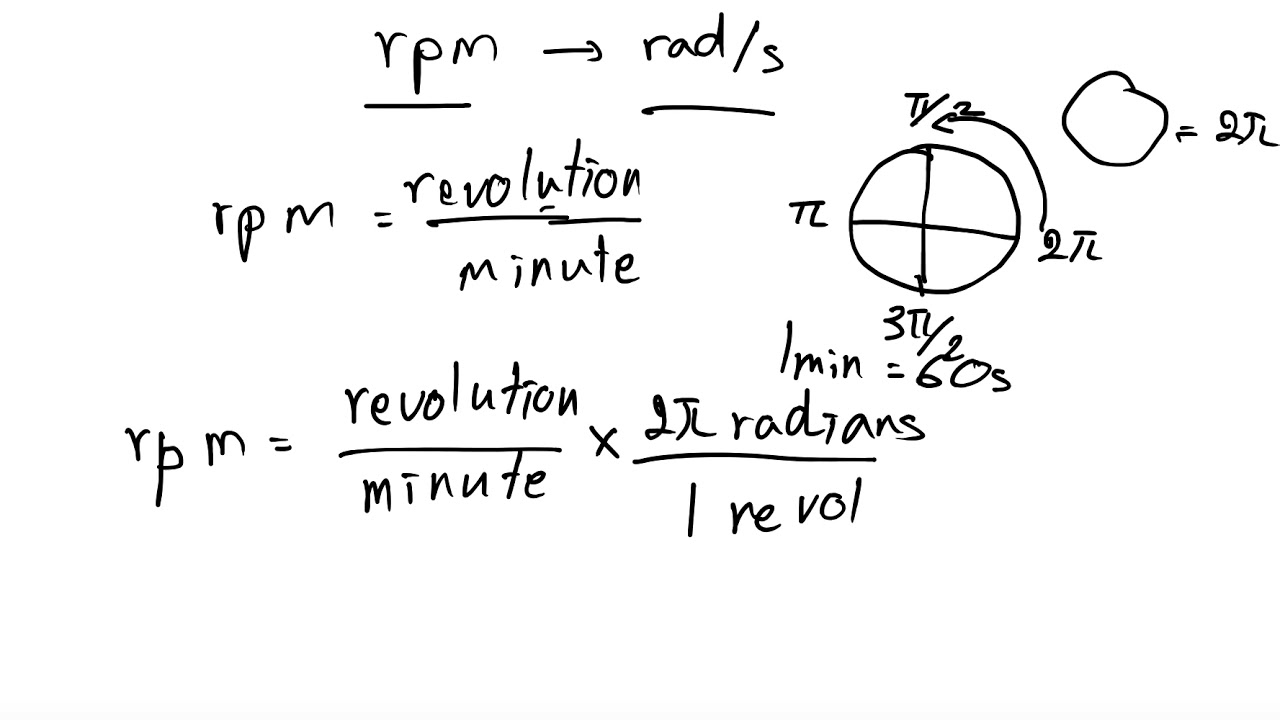Convert RPM to Rad/s How many RPM is 1 rad/sec? The answer is 9.5492965964254. We assume you are converting between RPM and radian/second. You can view more details on each measurement unit:

The SI-derived unit for frequency is the hertz. 1 hertz is equal to 60 RPM, or 6.2831853 rad/sec.
Note that rounding errors may occur, so always check the results. Use this page to learn how to convert between RPM and radians/second. Type in your own numbers in the form to convert the units!

Revolutions per second=60 second/minute rpm## Quick conversion chart of RPM to rad/sec

Revolutions
(rpm)
Angular Velocity
0 0.0 0
10 1.0 60
20 2.1 120
30 3.1 180
40 4.2 240
50 5.2 300
60 6.3 360
70 7.3 420
80 8.4 480
90 9.4 540
100 10.5 600

Convert MM to IN

#### FAQ

How do you convert rpm to rad s?

How do you convert rads s to RPM?

How do you convert rpm to meters per minute?

How do I calculate RPM?

How do you calculate rpm torque?

What is the formula for calculating torque?

How do we calculate torque?

How much torque does a 1 hp motor have?

Categories CC